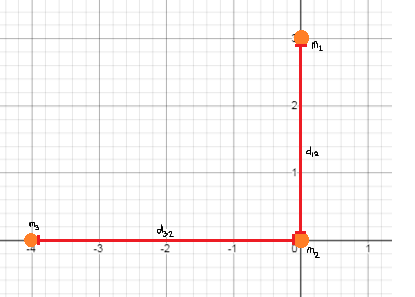# Three uniform spheres of masses m_1 = 3.50 \space kg, m_2 = 4.00 \space kg and m_3 = 5.00 \space...

## Question:

Three uniform spheres of masses {eq}m_1 = 3.50 \space kg, m_2 = 4.00 \space kg {/eq} and {eq}m_3 = 5.00 \space kg {/eq} are placed in an {eq}xy {/eq} plane at locations {eq}(0, +3.00 \space m), (0, 0) {/eq} and {eq}(-4.00 \space m, 0) {/eq} respectively. Calculate the resultant gravitational force on mass {eq}m_2 {/eq}, assuming the spheres are isolated from the rest of the Universe.

## Net Gravitational Force:

The net gravitational force on an object is determined by taking the sum of all the individual gravitational forces acting on it. The individual gravitational force is derived from the product of the two objects' masses and the inverse of the square of their distances.

Become a Study.com member to unlock this answer! Create your account

The spheres are arranged as described below:The individual gravitational...The Effects of Mass & Distance on Gravity

from

Chapter 5 / Lesson 13
39K

Does gravity have you down? Well, you are not alone. Gravity is the force that pulls objects with mass toward each other. It is what holds you to the earth. Learn how the strength of gravity is affected by mass and distance.